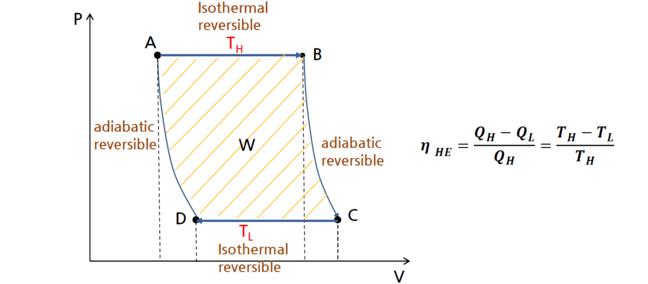3.11

# Heat engine P-V diagram

1. We have represented the heat engine cycle on a T-S diagram. Here we can express it on P-V diagram.
2. At the high temperature source, the boiler, the fluid experience isothermal phase change from liquid to vapor. This vaporization process happens at constant pressure increasing volume (from liquid to vapor)
3. At turbine, the gas expands adiabatically and reversibly. It follows equation of states for adiabatic, reversible process. If the gas behaves ideally the curve is $PV^Y=constant$.
4. At the low temperature source, the condenser, the fluid experience isothermal phase change from gas to liquid. This condensation process happens at constant pressure decreasing volume (from liquid to vapor). The pressure is lower than the initial pressure of the boiler since the phase transition temperature is lower ($\frac {dP}{dT}$ for two liquid-vapor equilibrium is positive).
5. At the compressor, the liquid is compressed adiabatically and reversibly increasing its pressure to the original point.

Structure of heat engine: P-V diagram